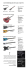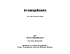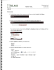# slides, pdf

## Transcription

slides, pdf
```CSR-2011
Kolmogorov complexity as a language
Alexander Shen
LIF CNRS, Marseille; on leave from
ИППИ РАН, Москва
.
.
.
.
.
.
Kolmogorov complexity
.
.
.
.
.
.
Kolmogorov complexity
I
A powerful tool
.
.
.
.
.
.
Kolmogorov complexity
I
A powerful tool
I
Just a way to reformulate arguments
.
.
.
.
.
.
Kolmogorov complexity
I
A powerful tool
I
Just a way to reformulate arguments
I
three languages: combinatorial/algorithmic/probabilistic
.
.
.
.
.
.
Kolmogorov complexity
I
A powerful tool
I
Just a way to reformulate arguments
I
three languages: combinatorial/algorithmic/probabilistic
.
.
.
.
.
.
Reminder and notation
.
.
.
.
.
.
Reminder and notation
I
K(x) = minimal length of a program that produces x
.
.
.
.
.
.
Reminder and notation
I
K(x) = minimal length of a program that produces x
I
KD (x) = min{|p| : D(p) = x}
.
.
.
.
.
.
Reminder and notation
I
K(x) = minimal length of a program that produces x
I
KD (x) = min{|p| : D(p) = x}
I
depends on the interpreter D
.
.
.
.
.
.
Reminder and notation
I
K(x) = minimal length of a program that produces x
I
KD (x) = min{|p| : D(p) = x}
I
depends on the interpreter D
I
optimal D makes it minimal up to O(1) additive term
.
.
.
.
.
.
Reminder and notation
I
K(x) = minimal length of a program that produces x
I
KD (x) = min{|p| : D(p) = x}
I
depends on the interpreter D
I
optimal D makes it minimal up to O(1) additive term
I
Variations:
.
.
.
.
.
.
Reminder and notation
I
K(x) = minimal length of a program that produces x
I
KD (x) = min{|p| : D(p) = x}
I
depends on the interpreter D
I
optimal D makes it minimal up to O(1) additive term
I
Variations:
x = string
x = preﬁx
of a sequence
p = string
plain
K(x), C(x)
decision
KR(x), KD(x)
p = preﬁx of a sequence
preﬁx
KP(x), K(x)
monotone
KM(x), Km(x)
.
.
.
.
.
.
Reminder and notation
I
K(x) = minimal length of a program that produces x
I
KD (x) = min{|p| : D(p) = x}
I
depends on the interpreter D
I
optimal D makes it minimal up to O(1) additive term
I
Variations:
x = string
x = preﬁx
of a sequence
p = string
plain
K(x), C(x)
decision
KR(x), KD(x)
p = preﬁx of a sequence
preﬁx
KP(x), K(x)
monotone
KM(x), Km(x)
Conditional complexity C(x|y):
minimal length of a program p : y 7→ x.
.
.
.
.
.
.
Reminder and notation
I
K(x) = minimal length of a program that produces x
I
KD (x) = min{|p| : D(p) = x}
I
depends on the interpreter D
I
optimal D makes it minimal up to O(1) additive term
I
Variations:
x = string
x = preﬁx
of a sequence
p = string
plain
K(x), C(x)
decision
KR(x), KD(x)
p = preﬁx of a sequence
preﬁx
KP(x), K(x)
monotone
KM(x), Km(x)
Conditional complexity C(x|y):
minimal length of a program p : y 7→ x.
There is also a priori probability (in two versions: discrete, on strings;
continuous, on preﬁxes)
.
.
.
.
.
.
Foundations of probability theory
.
.
.
.
.
.
Foundations of probability theory
I
Random object or random process?
.
.
.
.
.
.
Foundations of probability theory
I
Random object or random process?
I
“well shufﬂed deck of cards”: any meaning?
.
.
.
.
.
.
Foundations of probability theory
I
Random object or random process?
I
“well shufﬂed deck of cards”: any meaning?
[xkcd cartoon]
.
.
.
.
.
.
Foundations of probability theory
I
Random object or random process?
I
“well shufﬂed deck of cards”: any meaning?
[xkcd cartoon]
I
randomness = incompressibility (maximal complexity)
.
.
.
.
.
.
Foundations of probability theory
I
Random object or random process?
I
“well shufﬂed deck of cards”: any meaning?
[xkcd cartoon]
I
randomness = incompressibility (maximal complexity)
I
ω = ω1 ω2 . . . is random iff KM(ω1 . . . ωn ) ≥ n − O(1)
.
.
.
.
.
.
Foundations of probability theory
I
Random object or random process?
I
“well shufﬂed deck of cards”: any meaning?
[xkcd cartoon]
I
randomness = incompressibility (maximal complexity)
I
ω = ω1 ω2 . . . is random iff KM(ω1 . . . ωn ) ≥ n − O(1)
I
Classical probability theory: random sequence satisﬁes the
Strong Law of Large Numbers with probability 1
.
.
.
.
.
.
Foundations of probability theory
I
Random object or random process?
I
“well shufﬂed deck of cards”: any meaning?
[xkcd cartoon]
I
randomness = incompressibility (maximal complexity)
I
ω = ω1 ω2 . . . is random iff KM(ω1 . . . ωn ) ≥ n − O(1)
I
Classical probability theory: random sequence satisﬁes the
Strong Law of Large Numbers with probability 1
I
Algorithmic version: every (algorithmically) random
sequence satisﬁes SLLN
.
.
.
.
.
.
Foundations of probability theory
I
Random object or random process?
I
“well shufﬂed deck of cards”: any meaning?
[xkcd cartoon]
I
randomness = incompressibility (maximal complexity)
I
ω = ω1 ω2 . . . is random iff KM(ω1 . . . ωn ) ≥ n − O(1)
I
Classical probability theory: random sequence satisﬁes the
Strong Law of Large Numbers with probability 1
I
Algorithmic version: every (algorithmically) random
sequence satisﬁes SLLN
I
algorithmic ⇒ classical: Martin-Löf random sequences form
a set of measure 1.
.
.
.
.
.
.
Sampling random strings (S.Aaronson)
.
.
.
.
.
.
Sampling random strings (S.Aaronson)
I
A device that (being switched on) produces N-bit string and
stops
.
.
.
.
.
.
Sampling random strings (S.Aaronson)
I
A device that (being switched on) produces N-bit string and
stops
I
“The device produces a random string”: what does it mean?
.
.
.
.
.
.
Sampling random strings (S.Aaronson)
I
A device that (being switched on) produces N-bit string and
stops
I
“The device produces a random string”: what does it mean?
I
classical: the output distribution is close to the uniform one
.
.
.
.
.
.
Sampling random strings (S.Aaronson)
I
A device that (being switched on) produces N-bit string and
stops
I
“The device produces a random string”: what does it mean?
I
classical: the output distribution is close to the uniform one
I
effective: with high probability the output string is
incompressible
.
.
.
.
.
.
Sampling random strings (S.Aaronson)
I
A device that (being switched on) produces N-bit string and
stops
I
“The device produces a random string”: what does it mean?
I
classical: the output distribution is close to the uniform one
I
effective: with high probability the output string is
incompressible
I
not equivalent if no assumptions about the device
.
.
.
.
.
.
Sampling random strings (S.Aaronson)
I
A device that (being switched on) produces N-bit string and
stops
I
“The device produces a random string”: what does it mean?
I
classical: the output distribution is close to the uniform one
I
effective: with high probability the output string is
incompressible
I
not equivalent if no assumptions about the device
I
but are related under some assumptions
.
.
.
.
.
.
Example: matrices without uniform minors
.
.
.
.
.
.
Example: matrices without uniform minors
I
k × k minor of n × n Boolean matrix: select k rows and k
columns
.
.
.
.
.
.
Example: matrices without uniform minors
I
k × k minor of n × n Boolean matrix: select k rows and k
columns
I
minor is uniform if it is all-0 or all-1.
I
claim: there is a n × n bit matrix without k × k uniform
minors for k = 3 log n.
.
.
.
.
.
.
Counting argument and complexity reformulation
.
.
.
.
.
.
Counting argument and complexity reformulation
I
≤ nk × nk positions of the minor [k = 3 log n]
.
.
.
.
.
.
Counting argument and complexity reformulation
I
≤ nk × nk positions of the minor [k = 3 log n]
I
2 types of uniform minors (0/1)
.
.
.
.
.
.
Counting argument and complexity reformulation
I
≤ nk × nk positions of the minor [k = 3 log n]
I
2 types of uniform minors (0/1)
I
2n
2 −k2
possibilities for the rest
.
.
.
.
.
.
Counting argument and complexity reformulation
I
≤ nk × nk positions of the minor [k = 3 log n]
I
2 types of uniform minors (0/1)
I
2n
2 −k2
I n2k
possibilities for the rest
2 −k2
× 2 × 2n
=
.
.
.
.
.
.
Counting argument and complexity reformulation
I
≤ nk × nk positions of the minor [k = 3 log n]
I
2 types of uniform minors (0/1)
I
2n
2 −k2
I n2k
possibilities for the rest
2 −k2
× 2 × 2n
2 −9 log2
= 2log n×2×3 log n+1+(n
.
n)
.
2
< 2n .
.
.
.
.
Counting argument and complexity reformulation
I
≤ nk × nk positions of the minor [k = 3 log n]
I
2 types of uniform minors (0/1)
I
2n
2 −k2
I n2k
I
possibilities for the rest
2 −k2
× 2 × 2n
2 −9 log2
= 2log n×2×3 log n+1+(n
n)
2
< 2n .
log n bits to specify a column or row: 2k log n bits in total
.
.
.
.
.
.
Counting argument and complexity reformulation
I
≤ nk × nk positions of the minor [k = 3 log n]
I
2 types of uniform minors (0/1)
I
2n
2 −k2
I n2k
possibilities for the rest
2 −k2
× 2 × 2n
2 −9 log2
= 2log n×2×3 log n+1+(n
n)
2
< 2n .
I
log n bits to specify a column or row: 2k log n bits in total
I
one additional bit to specify the type of minor (0/1)
.
.
.
.
.
.
Counting argument and complexity reformulation
I
≤ nk × nk positions of the minor [k = 3 log n]
I
2 types of uniform minors (0/1)
I
2n
2 −k2
I n2k
possibilities for the rest
2 −k2
× 2 × 2n
2 −9 log2
= 2log n×2×3 log n+1+(n
n)
2
< 2n .
I
log n bits to specify a column or row: 2k log n bits in total
I
one additional bit to specify the type of minor (0/1)
I
n2 − k2 bits to specify the rest of the matrix
.
.
.
.
.
.
Counting argument and complexity reformulation
I
≤ nk × nk positions of the minor [k = 3 log n]
I
2 types of uniform minors (0/1)
I
2n
2 −k2
I n2k
possibilities for the rest
2 −k2
× 2 × 2n
2 −9 log2
= 2log n×2×3 log n+1+(n
n)
2
< 2n .
I
log n bits to specify a column or row: 2k log n bits in total
I
one additional bit to specify the type of minor (0/1)
I
n2 − k2 bits to specify the rest of the matrix
I
2k log n + 1 + (n2 − k2 ) = 6 log2 n + 1 + (n2 − 9 log2 n) < n2 .
.
.
.
.
.
.
One-tape Turing machines
.
.
.
.
.
.
One-tape Turing machines
I
copying n-bit string on 1-tape TM requires Ω(n2 ) time
.
.
.
.
.
.
One-tape Turing machines
I
copying n-bit string on 1-tape TM requires Ω(n2 ) time
I
complexity version: if initially the tape was empty on the
right of the border, then after n steps the complexity of a
zone that is d cells far from the border is O(n/d).
K(u(t)) ≤ O(n/d)
.
.
.
.
.
.
One-tape Turing machines
I
copying n-bit string on 1-tape TM requires Ω(n2 ) time
I
complexity version: if initially the tape was empty on the
right of the border, then after n steps the complexity of a
zone that is d cells far from the border is O(n/d).
K(u(t)) ≤ O(n/d)
I proof: border guards in each cell of the border security zone write down the
contents of the head of TM; each of the records is enough to reconstruct u(t) so the
length of it should be Ω(K(u(t)); the sum of lengths does not exceed time
.
.
.
.
.
.
Everywhere complex sequences
.
.
.
.
.
.
Everywhere complex sequences
I
Random sequence has n-bit preﬁx of complexity n
.
.
.
.
.
.
Everywhere complex sequences
I
Random sequence has n-bit preﬁx of complexity n
I
but some factors (substrings) have small complexity
.
.
.
.
.
.
Everywhere complex sequences
I
Random sequence has n-bit preﬁx of complexity n
I
but some factors (substrings) have small complexity
I
Levin: there exist everywhere complex sequences: every
n-bit substring has complexity 0.99n − O(1)
.
.
.
.
.
.
Everywhere complex sequences
I
Random sequence has n-bit preﬁx of complexity n
I
but some factors (substrings) have small complexity
I
Levin: there exist everywhere complex sequences: every
n-bit substring has complexity 0.99n − O(1)
I
Combinatorial equivalent: Let F be a set of strings that has at
most 20.99n strings of length n. Then there is a sequence ω
s.t. all sufﬁciently long substrings of ω are not in F.
.
.
.
.
.
.
Everywhere complex sequences
I
Random sequence has n-bit preﬁx of complexity n
I
but some factors (substrings) have small complexity
I
Levin: there exist everywhere complex sequences: every
n-bit substring has complexity 0.99n − O(1)
I
Combinatorial equivalent: Let F be a set of strings that has at
most 20.99n strings of length n. Then there is a sequence ω
s.t. all sufﬁciently long substrings of ω are not in F.
I
combinatorial and complexity proofs not just translations of
each other (Lovasz lemma, Rumyantsev, Miller, Muchnik)
.
.
.
.
.
.
Gilbert-Varshamov complexity bound
.
.
.
.
.
.
Gilbert-Varshamov complexity bound
I
coding theory: how many n-bit strings x1 , . . . , xk one can ﬁnd
if Hamming distance between every two is at least d
.
.
.
.
.
.
Gilbert-Varshamov complexity bound
I
coding theory: how many n-bit strings x1 , . . . , xk one can ﬁnd
if Hamming distance between every two is at least d
I
lower bound (Gilbert–Varshamov)
.
.
.
.
.
.
Gilbert-Varshamov complexity bound
I
coding theory: how many n-bit strings x1 , . . . , xk one can ﬁnd
if Hamming distance between every two is at least d
I
lower bound (Gilbert–Varshamov)
I
then < d/2 changed bits are harmless
.
.
.
.
.
.
Gilbert-Varshamov complexity bound
I
coding theory: how many n-bit strings x1 , . . . , xk one can ﬁnd
if Hamming distance between every two is at least d
I
lower bound (Gilbert–Varshamov)
I
then < d/2 changed bits are harmless
I
but bit insertion or deletions could be
.
.
.
.
.
.
Gilbert-Varshamov complexity bound
I
coding theory: how many n-bit strings x1 , . . . , xk one can ﬁnd
if Hamming distance between every two is at least d
I
lower bound (Gilbert–Varshamov)
I
then < d/2 changed bits are harmless
I
but bit insertion or deletions could be
I
general requirement: C(xi |xj ) ≥ d
.
.
.
.
.
.
Gilbert-Varshamov complexity bound
I
coding theory: how many n-bit strings x1 , . . . , xk one can ﬁnd
if Hamming distance between every two is at least d
I
lower bound (Gilbert–Varshamov)
I
then < d/2 changed bits are harmless
I
but bit insertion or deletions could be
I
general requirement: C(xi |xj ) ≥ d
I
generalization of GV bound: d-separated family of size
Ω(2n−d )
.
.
.
.
.
.
Inequalities for complexities and combinatorial
interpretation
.
.
.
.
.
.
Inequalities for complexities and combinatorial
interpretation
I
C(x, y) ≤ C(x) + C(y|x) + O(log)
.
.
.
.
.
.
Inequalities for complexities and combinatorial
interpretation
I
C(x, y) ≤ C(x) + C(y|x) + O(log)
.
.
.
.
.
.
Inequalities for complexities and combinatorial
interpretation
I
C(x, y) ≤ C(x) + C(y|x) + O(log)
I
C(x, y) ≥ C(x) + C(y|x) + O(log)
.
.
.
.
.
.
Inequalities for complexities and combinatorial
interpretation
I
C(x, y) ≤ C(x) + C(y|x) + O(log)
I
C(x, y) ≥ C(x) + C(y|x) + O(log)
I
C(x, y) < k + l ⇒ C(x) < k + O(log) or C(y|x) < l + O(log)
.
.
.
.
.
.
Inequalities for complexities and combinatorial
interpretation
I
C(x, y) ≤ C(x) + C(y|x) + O(log)
I
C(x, y) ≥ C(x) + C(y|x) + O(log)
I
C(x, y) < k + l ⇒ C(x) < k + O(log) or C(y|x) < l + O(log)
I
every set A of size < 2k+l can be split into two parts
A = A1 ∪ A2 such that w(A1 ) ≤ 2k and h(A2 ) ≤ 2l
.
.
.
.
.
.
One more inequality
.
.
.
.
.
.
One more inequality
I
2C(x, y, z) ≤ C(x, y) + C(y, z) + C(x, z)
.
.
.
.
.
.
One more inequality
I
2C(x, y, z) ≤ C(x, y) + C(y, z) + C(x, z)
I
V 2 ≤ S1 × S2 × S3
.
.
.
.
.
.
One more inequality
I
2C(x, y, z) ≤ C(x, y) + C(y, z) + C(x, z)
I
V 2 ≤ S1 × S2 × S3
I
Also for Shannon entropies; special case of Shearer lemma
.
.
.
.
.
.
Common information and graph minors
.
.
.
.
.
.
Common information and graph minors
I
mutual information: I(a : b) = C(a) + C(b) − C(a, b)
.
.
.
.
.
.
Common information and graph minors
I
mutual information: I(a : b) = C(a) + C(b) − C(a, b)
I
common information:
.
.
.
.
.
.
Common information and graph minors
I
mutual information: I(a : b) = C(a) + C(b) − C(a, b)
I
common information:
I
combinatorial: graph minors
can the graph be covered by 2δ minors of size 2α−δ × 2β−δ ?
.
.
.
.
.
.
Almost uniform sets
.
.
.
.
.
.
Almost uniform sets
I
nonuniformity= (maximal section)/(average section)
.
.
.
.
.
.
Almost uniform sets
I
nonuniformity= (maximal section)/(average section)
I
Theorem: every set of N elements can be represented as
union of polylog(N) sets whose nonuniformity is polylog(N).
.
.
.
.
.
.
Almost uniform sets
I
nonuniformity= (maximal section)/(average section)
I
Theorem: every set of N elements can be represented as
union of polylog(N) sets whose nonuniformity is polylog(N).
I
multidimensional version
.
.
.
.
.
.
Almost uniform sets
I
nonuniformity= (maximal section)/(average section)
I
Theorem: every set of N elements can be represented as
union of polylog(N) sets whose nonuniformity is polylog(N).
I
multidimensional version
I
how to construct parts using Kolmogorov complexity: take
strings with given complexity bounds
.
.
.
.
.
.
Almost uniform sets
I
nonuniformity= (maximal section)/(average section)
I
Theorem: every set of N elements can be represented as
union of polylog(N) sets whose nonuniformity is polylog(N).
I
multidimensional version
I
how to construct parts using Kolmogorov complexity: take
strings with given complexity bounds
I
so simple that it is not clear what is the combinatorial
translation
.
.
.
.
.
.
Almost uniform sets
I
nonuniformity= (maximal section)/(average section)
I
Theorem: every set of N elements can be represented as
union of polylog(N) sets whose nonuniformity is polylog(N).
I
multidimensional version
I
how to construct parts using Kolmogorov complexity: take
strings with given complexity bounds
I
so simple that it is not clear what is the combinatorial
translation
I
but combinatorial argument exists (and gives even a stronger
result)
.
.
.
.
.
.
Shannon coding theorem
.
.
.
.
.
.
Shannon coding theorem
I
ξ is a random variable; k values, probabilities p1 , . . . , pk
.
.
.
.
.
.
Shannon coding theorem
I
ξ is a random variable; k values, probabilities p1 , . . . , pk
I
ξ N : N independent trials of ξ
.
.
.
.
.
.
Shannon coding theorem
I
ξ is a random variable; k values, probabilities p1 , . . . , pk
I
ξ N : N independent trials of ξ
I
Shannon’s informal question: how many bits are needed to
encode a “typical” value of ξ N ?
.
.
.
.
.
.
Shannon coding theorem
I
ξ is a random variable; k values, probabilities p1 , . . . , pk
I
ξ N : N independent trials of ξ
I
Shannon’s informal question: how many bits are needed to
encode a “typical” value of ξ N ?
I
H(ξ) = p1 log(1/p1 ) + . . . + pn log(1/pn ).
.
.
.
.
.
.
Shannon coding theorem
I
ξ is a random variable; k values, probabilities p1 , . . . , pk
I
ξ N : N independent trials of ξ
I
Shannon’s informal question: how many bits are needed to
encode a “typical” value of ξ N ?
I
H(ξ) = p1 log(1/p1 ) + . . . + pn log(1/pn ).
I
formal statement is a bit complicated
.
.
.
.
.
.
Shannon coding theorem
I
ξ is a random variable; k values, probabilities p1 , . . . , pk
I
ξ N : N independent trials of ξ
I
Shannon’s informal question: how many bits are needed to
encode a “typical” value of ξ N ?
I
H(ξ) = p1 log(1/p1 ) + . . . + pn log(1/pn ).
I
formal statement is a bit complicated
I
Complexity version: with high probablity the value of ξ N has
complexity close to NH(ξ).
.
.
.
.
.
.
Complexity, entropy and group size
.
.
.
.
.
.
Complexity, entropy and group size
I
2C(x, y, z) ≤ C(x, y) + C(y, z) + C(x, z) + O(log)
.
.
.
.
.
.
Complexity, entropy and group size
I
2C(x, y, z) ≤ C(x, y) + C(y, z) + C(x, z) + O(log)
I
The same for entropy:
2H(ξ, η, τ ) ≤ H(ξ, η) + H(ξ, τ ) + H(η, τ )
.
.
.
.
.
.
Complexity, entropy and group size
I
2C(x, y, z) ≤ C(x, y) + C(y, z) + C(x, z) + O(log)
I
The same for entropy:
2H(ξ, η, τ ) ≤ H(ξ, η) + H(ξ, τ ) + H(η, τ )
I
…and even for the sizes of subgroups U, V, W of some ﬁnite
group G: 2 log(|G|/|U ∩ V ∩ W|) ≤
log(|G|/|U ∩ V|) + log(|G|/|U ∩ W|) + log(|G|/|V ∩ W|).
.
.
.
.
.
.
Complexity, entropy and group size
I
2C(x, y, z) ≤ C(x, y) + C(y, z) + C(x, z) + O(log)
I
The same for entropy:
2H(ξ, η, τ ) ≤ H(ξ, η) + H(ξ, τ ) + H(η, τ )
I
…and even for the sizes of subgroups U, V, W of some ﬁnite
group G: 2 log(|G|/|U ∩ V ∩ W|) ≤
log(|G|/|U ∩ V|) + log(|G|/|U ∩ W|) + log(|G|/|V ∩ W|).
I
in all three cases inequalities are the same (Romashchenko,
Chan, Yeung)
.
.
.
.
.
.
Complexity, entropy and group size
I
2C(x, y, z) ≤ C(x, y) + C(y, z) + C(x, z) + O(log)
I
The same for entropy:
2H(ξ, η, τ ) ≤ H(ξ, η) + H(ξ, τ ) + H(η, τ )
I
…and even for the sizes of subgroups U, V, W of some ﬁnite
group G: 2 log(|G|/|U ∩ V ∩ W|) ≤
log(|G|/|U ∩ V|) + log(|G|/|U ∩ W|) + log(|G|/|V ∩ W|).
I
in all three cases inequalities are the same (Romashchenko,
Chan, Yeung)
I
some of them are quite strange:
I(a : b) ≤
≤ I(a : b|c)+I(a : b|d)+I(c : d)+I(a : b|e)+I(a : e|b)+I(b : e|a)
.
.
.
.
.
.
Complexity, entropy and group size
I
2C(x, y, z) ≤ C(x, y) + C(y, z) + C(x, z) + O(log)
I
The same for entropy:
2H(ξ, η, τ ) ≤ H(ξ, η) + H(ξ, τ ) + H(η, τ )
I
…and even for the sizes of subgroups U, V, W of some ﬁnite
group G: 2 log(|G|/|U ∩ V ∩ W|) ≤
log(|G|/|U ∩ V|) + log(|G|/|U ∩ W|) + log(|G|/|V ∩ W|).
I
in all three cases inequalities are the same (Romashchenko,
Chan, Yeung)
I
some of them are quite strange:
I(a : b) ≤
≤ I(a : b|c)+I(a : b|d)+I(c : d)+I(a : b|e)+I(a : e|b)+I(b : e|a)
I
Related to Romashchenko’s theorem: if three last terms are
zeros, one can extract common information from a, b, e.
.
.
.
.
.
.
Muchnik and Slepian–Wolf
.
.
.
.
.
.
Muchnik and Slepian–Wolf
I
a, b: two strings
.
.
.
.
.
.
Muchnik and Slepian–Wolf
I
a, b: two strings
I
we look for a program p that maps a to b
.
.
.
.
.
.
Muchnik and Slepian–Wolf
I
a, b: two strings
I
we look for a program p that maps a to b
I
by deﬁnition C(p) is at least C(b|a) but could be higher
.
.
.
.
.
.
Muchnik and Slepian–Wolf
I
a, b: two strings
I
we look for a program p that maps a to b
I
by deﬁnition C(p) is at least C(b|a) but could be higher
I
there exist p : a 7→ b that is simple relative to b, e.g., “map
everything to b”
.
.
.
.
.
.
Muchnik and Slepian–Wolf
I
a, b: two strings
I
we look for a program p that maps a to b
I
by deﬁnition C(p) is at least C(b|a) but could be higher
I
there exist p : a 7→ b that is simple relative to b, e.g., “map
everything to b”
I
Muchnik theorem: it is possible to combine these two
conditions: there exists p : a 7→ b such that C(p) ≈ C(b|a) and
C(p|b) ≈ 0
.
.
.
.
.
.
Muchnik and Slepian–Wolf
I
a, b: two strings
I
we look for a program p that maps a to b
I
by deﬁnition C(p) is at least C(b|a) but could be higher
I
there exist p : a 7→ b that is simple relative to b, e.g., “map
everything to b”
I
Muchnik theorem: it is possible to combine these two
conditions: there exists p : a 7→ b such that C(p) ≈ C(b|a) and
C(p|b) ≈ 0
I
information theory analog: Wolf–Slepian
.
.
.
.
.
.
Muchnik and Slepian–Wolf
I
a, b: two strings
I
we look for a program p that maps a to b
I
by deﬁnition C(p) is at least C(b|a) but could be higher
I
there exist p : a 7→ b that is simple relative to b, e.g., “map
everything to b”
I
Muchnik theorem: it is possible to combine these two
conditions: there exists p : a 7→ b such that C(p) ≈ C(b|a) and
C(p|b) ≈ 0
I
information theory analog: Wolf–Slepian
I
similar technique was developed by Fortnow and Laplante
(randomness extractors)
.
.
.
.
.
.
Muchnik and Slepian–Wolf
I
a, b: two strings
I
we look for a program p that maps a to b
I
by deﬁnition C(p) is at least C(b|a) but could be higher
I
there exist p : a 7→ b that is simple relative to b, e.g., “map
everything to b”
I
Muchnik theorem: it is possible to combine these two
conditions: there exists p : a 7→ b such that C(p) ≈ C(b|a) and
C(p|b) ≈ 0
I
information theory analog: Wolf–Slepian
I
similar technique was developed by Fortnow and Laplante
(randomness extractors)
I
(Romashchenko, Musatov): how to use explicit extractors and
derandomization to get space-bounded versions
.
.
.
.
.
.
Computability theory: simple sets
.
.
.
.
.
.
Computability theory: simple sets
I
Simple set: enumerable set with inﬁnite complement, but no
algorithm can generate inﬁnitely many elements from the
complement
.
.
.
.
.
.
Computability theory: simple sets
I
Simple set: enumerable set with inﬁnite complement, but no
algorithm can generate inﬁnitely many elements from the
complement
I
Construction using Kolmogorov complexity: a simple string x
has C(x) ≤ |x|/2.
.
.
.
.
.
.
Computability theory: simple sets
I
Simple set: enumerable set with inﬁnite complement, but no
algorithm can generate inﬁnitely many elements from the
complement
I
Construction using Kolmogorov complexity: a simple string x
has C(x) ≤ |x|/2.
I
Most strings are not simple ⇒ inﬁnite complement
.
.
.
.
.
.
Computability theory: simple sets
I
Simple set: enumerable set with inﬁnite complement, but no
algorithm can generate inﬁnitely many elements from the
complement
I
Construction using Kolmogorov complexity: a simple string x
has C(x) ≤ |x|/2.
I
Most strings are not simple ⇒ inﬁnite complement
I
Let x1 , x2 , . . . be a computable sequence of different
non-simple strings
.
.
.
.
.
.
Computability theory: simple sets
I
Simple set: enumerable set with inﬁnite complement, but no
algorithm can generate inﬁnitely many elements from the
complement
I
Construction using Kolmogorov complexity: a simple string x
has C(x) ≤ |x|/2.
I
Most strings are not simple ⇒ inﬁnite complement
I
Let x1 , x2 , . . . be a computable sequence of different
non-simple strings
I
May assume wlog that |xi | > i and therefore C(xi ) > i/2
.
.
.
.
.
.
Computability theory: simple sets
I
Simple set: enumerable set with inﬁnite complement, but no
algorithm can generate inﬁnitely many elements from the
complement
I
Construction using Kolmogorov complexity: a simple string x
has C(x) ≤ |x|/2.
I
Most strings are not simple ⇒ inﬁnite complement
I
Let x1 , x2 , . . . be a computable sequence of different
non-simple strings
I
May assume wlog that |xi | > i and therefore C(xi ) > i/2
I
but to specify xi we need O(log i) bits only
.
.
.
.
.
.
Computability theory: simple sets
I
Simple set: enumerable set with inﬁnite complement, but no
algorithm can generate inﬁnitely many elements from the
complement
I
Construction using Kolmogorov complexity: a simple string x
has C(x) ≤ |x|/2.
I
Most strings are not simple ⇒ inﬁnite complement
I
Let x1 , x2 , . . . be a computable sequence of different
non-simple strings
I
May assume wlog that |xi | > i and therefore C(xi ) > i/2
I
but to specify xi we need O(log i) bits only
I
“Minimal integer that cannot be described in ten English
words” (Berry)
.
.
.
.
.
.
Lower semicomputable random reals
.
.
.
.
.
.
Lower semicomputable random reals
I
∑
ai computable converging series with rational terms
.
.
.
.
.
.
Lower semicomputable random reals
I
I
∑
ai computable converging series with rational terms
∑
is α =
ai computable (ε → ε-approximation)?
.
.
.
.
.
.
Lower semicomputable random reals
I
∑
I
ai computable converging series with rational terms
∑
is α =
ai computable (ε → ε-approximation)?
I
not necessarily (Specker example)
.
.
.
.
.
.
Lower semicomputable random reals
I
∑
I
ai computable converging series with rational terms
∑
is α =
ai computable (ε → ε-approximation)?
I
not necessarily (Specker example)
I
lower semicomputable reals
.
.
.
.
.
.
Lower semicomputable random reals
I
∑
I
ai computable converging series with rational terms
∑
is α =
ai computable (ε → ε-approximation)?
I
not necessarily (Specker example)
I
lower semicomputable reals
I
Solovay classiﬁcation: α β if ε-approximation to β can be
effectively converted to O(ε)-approximation to α
.
.
.
.
.
.
Lower semicomputable random reals
I
∑
I
ai computable converging series with rational terms
∑
is α =
ai computable (ε → ε-approximation)?
I
not necessarily (Specker example)
I
lower semicomputable reals
I
Solovay classiﬁcation: α β if ε-approximation to β can be
effectively converted to O(ε)-approximation to α
I
There are maximal elements
.
.
.
.
.
.
Lower semicomputable random reals
I
∑
I
ai computable converging series with rational terms
∑
is α =
ai computable (ε → ε-approximation)?
I
not necessarily (Specker example)
I
lower semicomputable reals
I
Solovay classiﬁcation: α β if ε-approximation to β can be
effectively converted to O(ε)-approximation to α
I
There are maximal elements = random lower
semicomputable reals
.
.
.
.
.
.
Lower semicomputable random reals
I
∑
I
ai computable converging series with rational terms
∑
is α =
ai computable (ε → ε-approximation)?
I
not necessarily (Specker example)
I
lower semicomputable reals
I
Solovay classiﬁcation: α β if ε-approximation to β can be
effectively converted to O(ε)-approximation to α
I
There are maximal elements = random lower
semicomputable reals
I
= slowly converging series
.
.
.
.
.
.
Lower semicomputable random reals
I
∑
I
ai computable converging series with rational terms
∑
is α =
ai computable (ε → ε-approximation)?
I
not necessarily (Specker example)
I
lower semicomputable reals
I
Solovay classiﬁcation: α β if ε-approximation to β can be
effectively converted to O(ε)-approximation to α
I
There are maximal elements = random lower
semicomputable reals
I
= slowly converging series
I
modulus of convergence: ε 7→ N(ε) = how many terms are
needed for ε-precision
.
.
.
.
.
.
Lower semicomputable random reals
I
∑
I
ai computable converging series with rational terms
∑
is α =
ai computable (ε → ε-approximation)?
I
not necessarily (Specker example)
I
lower semicomputable reals
I
Solovay classiﬁcation: α β if ε-approximation to β can be
effectively converted to O(ε)-approximation to α
I
There are maximal elements = random lower
semicomputable reals
I
= slowly converging series
I
modulus of convergence: ε 7→ N(ε) = how many terms are
needed for ε-precision
I
maximal elements: N(2−n ) > BP(n − O(1)), where BP(k) is
the maximal integer whose preﬁx complexity is k or less.
.
.
.
.
.
.
Lovasz local lemma: constructive proof
.
.
.
.
.
.
Lovasz local lemma: constructive proof
I
CNF: (a ∧ ¬b ∧ . . .) ∨ (¬c ∧ e ∧ . . .) ∨ . . .
.
.
.
.
.
.
Lovasz local lemma: constructive proof
I
CNF: (a ∧ ¬b ∧ . . .) ∨ (¬c ∧ e ∧ . . .) ∨ . . .
I
neighbors: clauses having common variables
.
.
.
.
.
.
Lovasz local lemma: constructive proof
I
CNF: (a ∧ ¬b ∧ . . .) ∨ (¬c ∧ e ∧ . . .) ∨ . . .
I
neighbors: clauses having common variables
I
several clauses with k literals in each
.
.
.
.
.
.
Lovasz local lemma: constructive proof
I
CNF: (a ∧ ¬b ∧ . . .) ∨ (¬c ∧ e ∧ . . .) ∨ . . .
I
neighbors: clauses having common variables
I
several clauses with k literals in each
I
each clause has o(2k ) neighbors
.
.
.
.
.
.
Lovasz local lemma: constructive proof
I
CNF: (a ∧ ¬b ∧ . . .) ∨ (¬c ∧ e ∧ . . .) ∨ . . .
I
neighbors: clauses having common variables
I
several clauses with k literals in each
I
each clause has o(2k ) neighbors
I
⇒ CNF is satisﬁable
.
.
.
.
.
.
Lovasz local lemma: constructive proof
I
CNF: (a ∧ ¬b ∧ . . .) ∨ (¬c ∧ e ∧ . . .) ∨ . . .
I
neighbors: clauses having common variables
I
several clauses with k literals in each
I
each clause has o(2k ) neighbors
I
⇒ CNF is satisﬁable
I
Non-constructive proof: lower bound for probability, Lovasz
local lemma
.
.
.
.
.
.
Lovasz local lemma: constructive proof
I
CNF: (a ∧ ¬b ∧ . . .) ∨ (¬c ∧ e ∧ . . .) ∨ . . .
I
neighbors: clauses having common variables
I
several clauses with k literals in each
I
each clause has o(2k ) neighbors
I
⇒ CNF is satisﬁable
I
Non-constructive proof: lower bound for probability, Lovasz
local lemma
I
Naïve algorithm: just resample false clause (while they exist)
.
.
.
.
.
.
Lovasz local lemma: constructive proof
I
CNF: (a ∧ ¬b ∧ . . .) ∨ (¬c ∧ e ∧ . . .) ∨ . . .
I
neighbors: clauses having common variables
I
several clauses with k literals in each
I
each clause has o(2k ) neighbors
I
⇒ CNF is satisﬁable
I
Non-constructive proof: lower bound for probability, Lovasz
local lemma
I
Naïve algorithm: just resample false clause (while they exist)
I
Recent breakthrough (Moser): this algorithm with high
probability terminates quickly
.
.
.
.
.
.
Lovasz local lemma: constructive proof
I
CNF: (a ∧ ¬b ∧ . . .) ∨ (¬c ∧ e ∧ . . .) ∨ . . .
I
neighbors: clauses having common variables
I
several clauses with k literals in each
I
each clause has o(2k ) neighbors
I
⇒ CNF is satisﬁable
I
Non-constructive proof: lower bound for probability, Lovasz
local lemma
I
Naïve algorithm: just resample false clause (while they exist)
I
Recent breakthrough (Moser): this algorithm with high
probability terminates quickly
I
Explanation: if not, the sequence of resampled clauses
would encode the random bits used in resampling making
them compressible
.
.
.
.
.
.
Berry, Gödel, Chaitin, Raz
.
.
.
.
.
.
Berry, Gödel, Chaitin, Raz
I
There are only ﬁnitely many strings of complexity < n
.
.
.
.
.
.
Berry, Gödel, Chaitin, Raz
I
There are only ﬁnitely many strings of complexity < n
I
for all strings (except ﬁnitely many ones) x the statement
K(x) > n is true
.
.
.
.
.
.
Berry, Gödel, Chaitin, Raz
I
There are only ﬁnitely many strings of complexity < n
I
for all strings (except ﬁnitely many ones) x the statement
K(x) > n is true
I
Can all true statements of this form be provable?
.
.
.
.
.
.
Berry, Gödel, Chaitin, Raz
I
There are only ﬁnitely many strings of complexity < n
I
for all strings (except ﬁnitely many ones) x the statement
K(x) > n is true
I
Can all true statements of this form be provable?
I
No, otherwise we could effectively generate string of
complexity > n by enumerating all proofs
.
.
.
.
.
.
Berry, Gödel, Chaitin, Raz
I
There are only ﬁnitely many strings of complexity < n
I
for all strings (except ﬁnitely many ones) x the statement
K(x) > n is true
I
Can all true statements of this form be provable?
I
No, otherwise we could effectively generate string of
complexity > n by enumerating all proofs
I
and get Berry’s paradox: the ﬁrst provable statement
C(x) > n for given n gives some x of complexity > n that can
be described by O(log n) bits
.
.
.
.
.
.
Berry, Gödel, Chaitin, Raz
I
There are only ﬁnitely many strings of complexity < n
I
for all strings (except ﬁnitely many ones) x the statement
K(x) > n is true
I
Can all true statements of this form be provable?
I
No, otherwise we could effectively generate string of
complexity > n by enumerating all proofs
I
and get Berry’s paradox: the ﬁrst provable statement
C(x) > n for given n gives some x of complexity > n that can
be described by O(log n) bits
I
(Gödel theorem in Chaitin form): There are only ﬁnitely many
n such that C(x) > n is provable for some x.
.
.
.
.
.
.
Berry, Gödel, Chaitin, Raz
I
There are only ﬁnitely many strings of complexity < n
I
for all strings (except ﬁnitely many ones) x the statement
K(x) > n is true
I
Can all true statements of this form be provable?
I
No, otherwise we could effectively generate string of
complexity > n by enumerating all proofs
I
and get Berry’s paradox: the ﬁrst provable statement
C(x) > n for given n gives some x of complexity > n that can
be described by O(log n) bits
I
(Gödel theorem in Chaitin form): There are only ﬁnitely many
n such that C(x) > n is provable for some x. (Note that
∃x C(x) > n is always provable!)
.
.
.
.
.
.
Berry, Gödel, Chaitin, Raz
I
There are only ﬁnitely many strings of complexity < n
I
for all strings (except ﬁnitely many ones) x the statement
K(x) > n is true
I
Can all true statements of this form be provable?
I
No, otherwise we could effectively generate string of
complexity > n by enumerating all proofs
I
and get Berry’s paradox: the ﬁrst provable statement
C(x) > n for given n gives some x of complexity > n that can
be described by O(log n) bits
I
(Gödel theorem in Chaitin form): There are only ﬁnitely many
n such that C(x) > n is provable for some x. (Note that
∃x C(x) > n is always provable!)
I
(Gödel second theorem, Kritchman–Raz proof): the
“unexpected test paradox” (a test will be given next week but
it won’t be known before the day of the test)
.
.
.
.
.
.
13th Hilbert’s problem
.
.
.
.
.
.
13th Hilbert’s problem
I
Function of ≥ 3 variables: e.g., solution of a polynomial of
degree 7 as function of its coefﬁcients
.
.
.
.
.
.
13th Hilbert’s problem
I
Function of ≥ 3 variables: e.g., solution of a polynomial of
degree 7 as function of its coefﬁcients
I
Is it possible to represent this function as a composition of
functions of at most 2 variables?
.
.
.
.
.
.
13th Hilbert’s problem
I
Function of ≥ 3 variables: e.g., solution of a polynomial of
degree 7 as function of its coefﬁcients
I
Is it possible to represent this function as a composition of
functions of at most 2 variables?
I
Yes, with weird functions (Cantor)
.
.
.
.
.
.
13th Hilbert’s problem
I
Function of ≥ 3 variables: e.g., solution of a polynomial of
degree 7 as function of its coefﬁcients
I
Is it possible to represent this function as a composition of
functions of at most 2 variables?
I
Yes, with weird functions (Cantor)
I
Yes, even with continuous functions (Kolmogorov, Arnold)
.
.
.
.
.
.
13th Hilbert’s problem
I
Function of ≥ 3 variables: e.g., solution of a polynomial of
degree 7 as function of its coefﬁcients
I
Is it possible to represent this function as a composition of
functions of at most 2 variables?
I
Yes, with weird functions (Cantor)
I
Yes, even with continuous functions (Kolmogorov, Arnold)
I
Circuit version: explicit function Bn × Bn × Bn → Bn
(polynomial circuit size?) that cannot be represented as
composition of O(1) functions Bn × Bn → Bn . Not known.
.
.
.
.
.
.
13th Hilbert’s problem
I
Function of ≥ 3 variables: e.g., solution of a polynomial of
degree 7 as function of its coefﬁcients
I
Is it possible to represent this function as a composition of
functions of at most 2 variables?
I
Yes, with weird functions (Cantor)
I
Yes, even with continuous functions (Kolmogorov, Arnold)
I
Circuit version: explicit function Bn × Bn × Bn → Bn
(polynomial circuit size?) that cannot be represented as
composition of O(1) functions Bn × Bn → Bn . Not known.
I
Kolmogorov complexity version: we have three strings a, b, c
on a blackboard. It is allowed to write (add) a new string if it
simple relative to two of the strings on the board. Which
strings we can obtain in O(1) steps? Only strings of small
complexity relative to a, b, c, but not all of them (for random
a, b, c)
.
.
.
.
.
.
Secret sharing
.
.
.
.
.
.
Secret sharing
I
secret s and three people; any two are able to reconstruct the
secret working together; but each one in isolation has no
.
.
.
.
.
.
Secret sharing
I
secret s and three people; any two are able to reconstruct the
secret working together; but each one in isolation has no
I
assume s ∈ F (a ﬁeld); take random a and tell the people a,
a + s and a + 2s (assuming 2 6= 0 in F)
.
.
.
.
.
.
Secret sharing
I
secret s and three people; any two are able to reconstruct the
secret working together; but each one in isolation has no
I
assume s ∈ F (a ﬁeld); take random a and tell the people a,
a + s and a + 2s (assuming 2 6= 0 in F)
I
other secret sharing schemes; how long should be secrets
(not understood)
.
.
.
.
.
.
Secret sharing
I
secret s and three people; any two are able to reconstruct the
secret working together; but each one in isolation has no
I
assume s ∈ F (a ﬁeld); take random a and tell the people a,
a + s and a + 2s (assuming 2 6= 0 in F)
I
other secret sharing schemes; how long should be secrets
(not understood)
I
Kolmogorov complexity settings: for a given s ﬁnd a, b, c
such that
C(s|a), C(s|b), C(s|c) ≈ C(s); C(s|a, b), C(s|a, c), C(s|b, c) ≈ 0
.
.
.
.
.
.
Secret sharing
I
secret s and three people; any two are able to reconstruct the
secret working together; but each one in isolation has no
I
assume s ∈ F (a ﬁeld); take random a and tell the people a,
a + s and a + 2s (assuming 2 6= 0 in F)
I
other secret sharing schemes; how long should be secrets
(not understood)
I
Kolmogorov complexity settings: for a given s ﬁnd a, b, c
such that
C(s|a), C(s|b), C(s|c) ≈ C(s); C(s|a, b), C(s|a, c), C(s|b, c) ≈ 0
I
Some relation between Kolmogorov and traditional settings
(Romashchenko, Kaced)
.
.
.
.
.
.
Quasi-cryptography
.
.
.
.
.
.
Quasi-cryptography
I
Alice has some information a
.
.
.
.
.
.
Quasi-cryptography
I
Alice has some information a
I
Bob wants to let her know some b
.
.
.
.
.
.
Quasi-cryptography
I
Alice has some information a
I
Bob wants to let her know some b
I
by sending some message f
.
.
.
.
.
.
Quasi-cryptography
I
Alice has some information a
I
Bob wants to let her know some b
I
by sending some message f
I
in such a way that Eve gets minimal information about b
.
.
.
.
.
.
Quasi-cryptography
I
Alice has some information a
I
Bob wants to let her know some b
I
by sending some message f
I
in such a way that Eve gets minimal information about b
I
Formally: for given a, b ﬁnd f such that C(b|a, f) ≈ 0 and
C(b|f) → max.
.
.
.
.
.
.
Quasi-cryptography
I
Alice has some information a
I
Bob wants to let her know some b
I
by sending some message f
I
in such a way that Eve gets minimal information about b
I
Formally: for given a, b ﬁnd f such that C(b|a, f) ≈ 0 and
C(b|f) → max.
I
Theorem (Muchnik): it is always possible to have
C(b|f) ≈ min(C(b), C(a))
.
.
.
.
.
.
Quasi-cryptography
I
Alice has some information a
I
Bob wants to let her know some b
I
by sending some message f
I
in such a way that Eve gets minimal information about b
I
Formally: for given a, b ﬁnd f such that C(b|a, f) ≈ 0 and
C(b|f) → max.
I
Theorem (Muchnik): it is always possible to have
C(b|f) ≈ min(C(b), C(a))
I
Full version: Eve knows some c and we want to send
message of a minimal possible length C(b|a)
.
.
.
.
.
.
Andrej Muchnik (1958-2007)
.
.
.
.
.
.
```

### Online Official Mail-In Order Form Instructions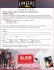### welcomeletter recruiting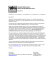### Sound Physics: The Piano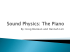### Min-ETune QUICK START GUIDE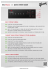### 29 SPECIALTY PENNANTS PRESTO INSTANT CANOPY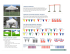### Basic Information - Tecnológico de Monterrey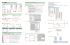### VST330 STRING SYNTHESIZER VSTi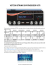### Current Steinberger guitar line-up (as of July 2015)